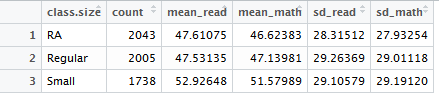# Project STAR (Student/Teacher Achievement Ratio)-R Assignment Sample

QUESTION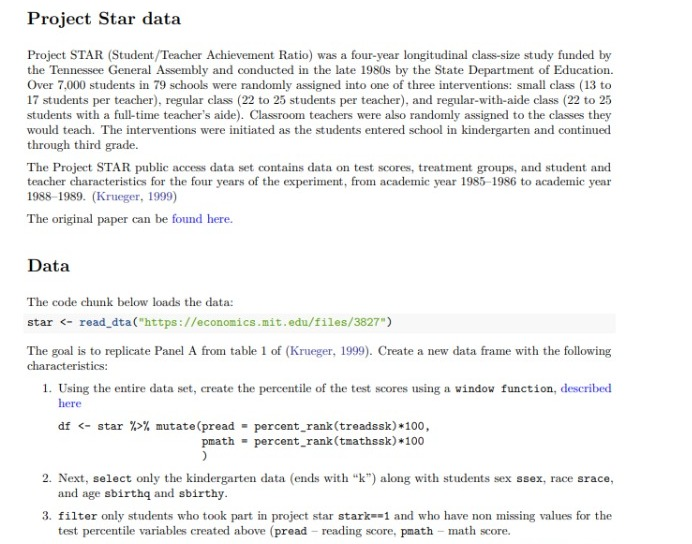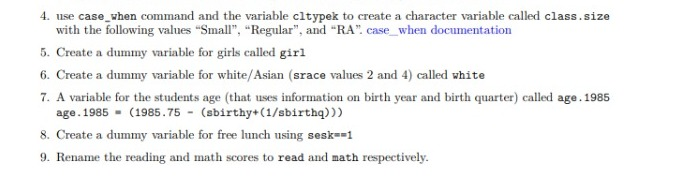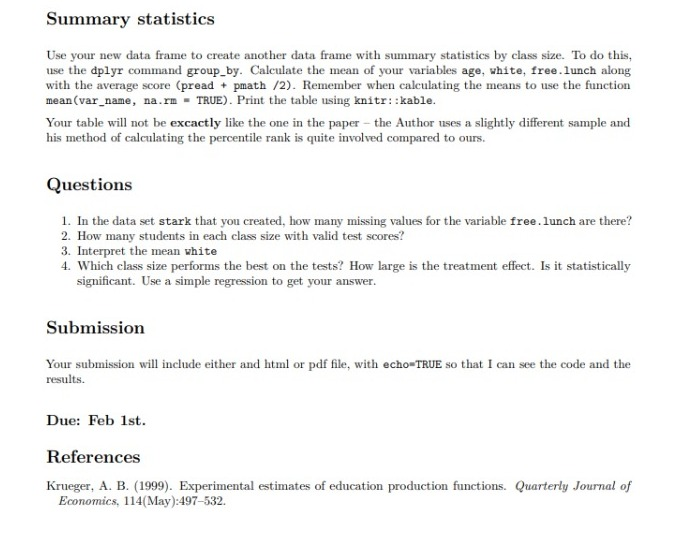Summary Statistics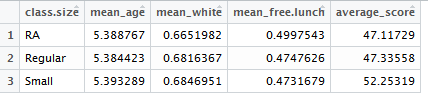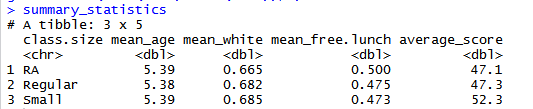Above is the summary statistics table according to the class size. In this table, we can see that the average age of students remains almost equal across the class. The average no of whites (white or Asian) is higher is Regular and small class sizes as compared to RA class size. Apart from this, the mean value of free lunch is higher in RA as compared to Regular and Small class sizes. This tells us about the correlation of size of class and the provision of free lunch. The average score of tests (read and math) is the highest for the “Small” class size than the other two. It can be implied that the class size impacts the score of the students, the significance of which has been checked further.

1. Ans. There are total 17 missing values for the variable free.lunch in the data frame stark.

2. Ans. The no of students in each class size with valid test scores are as follows: –

RA – 2043, Regular – 2005, Small – 1738

3. Ans. The mean value of variable white is 0.677, which shows that around two-third of the students are of either white or Asian race.

4. Ans. The smallest class size i.e. “Small” performs the best in the tests with their mean scores as 52.25. The treatment effect is not significantly large.

Null Hypothesis – There is no statistically significant difference in the test scores of the three classes.

Alternate Hypothesis – There is a statistically significant difference in the test scores of the three classes.